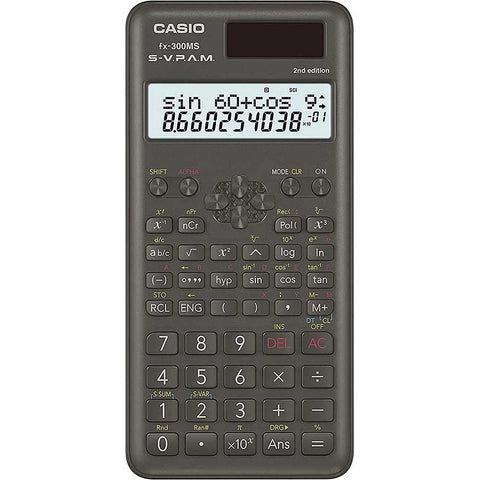# Casio FX300MS PLUS Scientific Calculator

Casio

• \$49.00

• 2-Line display
• 240 functions
• Multi-replay function
• Slide-on hard case included
• Supports Complex number calculations
• Backspace key
• Auto Replay function to check last equation
• Calculate Sine, Cosine, Tangent, and inverse
• Compute permutations and combinations
• Basic scientific, exponential, and trigonometric functions